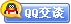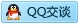##用户名 Email 自动登录 找回密码 密码 会员注册
 VIP会员，3年作业免费下 ！ 奥鹏作业，奥鹏毕业论文检测 新手作业下载教程，充值问题 没有找到答案，请在此处留言！ 2020年07月最新全国统考资料 投诉建议，加盟合作！奥鹏课程积分软件(ver:3.1)

# 大工12春《应用统计》在线作业3发表于 2012-5-3 21:50:48 | 显示全部楼层 |阅读模式大工12春《应用统计》在线作业3 试卷总分：100       测试时间：-- 判断题 单选题   一、判断题（共 10 道试题，共 40 分。）V 1.  方差刻画了随机变量的取值对于其数学期望的离散程度。 A. 错误 B. 正确       满分：4  分 2.  对于常数a,b，随机变量X，Y，有E（aX+bY）=aE(X)+bE(Y)。 A. 错误 B. 正确       满分：4  分 3.  若随机变量X，Y相互独立，则有D（X+Y）=D(X)+D(Y)。 A. 错误 B. 正确       满分：4  分 4.  对于X服从二项分布B（n,p）,则E(X)=p。 A. 错误 B. 正确       满分：4  分 5.  对于常数C，有E（C）=0。 A. 错误 B. 正确       满分：4  分 6.  若随机变量X，Y相互独立，则有E（XY）=E(X)E(Y)。 A. 错误 B. 正确       满分：4  分 7.  若随机变量X的取值比较集中，则D（X）较大。 A. 错误 B. 正确       满分：4  分 8.  对于常数C，有D（C）=0。 A. 错误 B. 正确       满分：4  分 9.  对于随机变量X，则有D（3X）=3D(X)。 A. 错误 B. 正确       满分：4  分 10.  数学期望描述随机变量取值的平均特征。 A. 错误 B. 正确       满分：4  分 谋学网 www.mouxue.com 二、单选题（共 10 道试题，共 60 分。）V 1.  对于随机变量X，Y，下列各式中不正确的是（）。 A. cov(X,Y)=E(XY)-E(X)E(Y) B. cov（aX，bY）=abcov（X，Y） C. 若X与Y相互独立，则cov(X,Y)=1 D. D(X+Y)=D(X)+D(Y)+2cov(X，Y)       满分：6  分 2.  对于随机变量X，Y有D（X）=4，D（Y）=9，cov(X,Y)=0.6,则相关系数ρxy=（）。 A. 0.6 B. 0.1 C. 0.2 D. 0.9       满分：6  分 3.  对于随机变量X服从正态分布，即X~N(0,4），则X的标准差是（）。 A. 0 B. 4 C. 2 D. 16       满分：6  分 4.  对于随机变量X，Y，下列各式中错误的是（）。 A. cov(X,Y)=cov（Y，X） B. cov（2X，Y）=2cov（X，Y） C. cov（X，X）=D（X） D. D（3X）=3D(X)       满分：6  分 5.  对于两个独立的随机变量X，Y服从正态分布，即X~N(4,1），Y~N(1,0.25），则D(2X+3Y)=（）。 A. 1.25 B. 6.25 C. 0.75 D. 2.75       满分：6  分 6.  对于两个独立的随机变量X，Y服从正态分布，即X~N(4,9），Y~N(1,4），则Z=X+Y服从（ ）分布。 A. Z~N(4,9） B. Z~N(3,5） C. Z~N(5,13） D. Z~N(5,5）       满分：6  分 7.  对于两个独立的随机变量X，Y服从正态分布，即X~N(4,9），Y~N(1,4）则，E(2X+3Y)=（）。 A. 9 B. 11 C. 13 D. 7       满分：6  分 8.  以下不属于正态分布概率密度曲线的特征是（）。 A. 标准差决定正态分布曲线的中心位置 B. 对称性 C. 集中性 D. 均匀变动性，即从均值所在处开始逐渐向两侧均匀下降       满分：6  分 9.  对于一般正态分布，如X~N(1,4)，则有关该正态分布的概率密度曲线的叙述不正确的是（ ）。 A. 该分布的概率密度函数曲线关于x=1对称 B. 在x=1处达到最大值 C. x轴为渐近线 D. 该概率密度函数曲线关于y轴对称       满分：6  分 10.  对于随机变量X服从正态分布，即X~N(4,9），则E（X）+D（X）=（）。 A. 13 B. 5 C. 11 D. 7       满分：6  分

### 本帖子中包含更多资源

x
 本版积分规则 回帖并转播 回帖后跳转到最后一页客服一客服二客服三客服四微信客服扫一扫